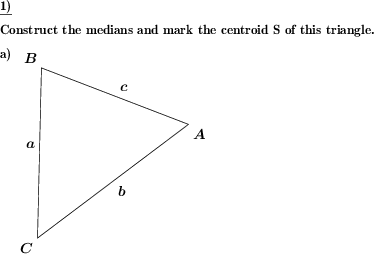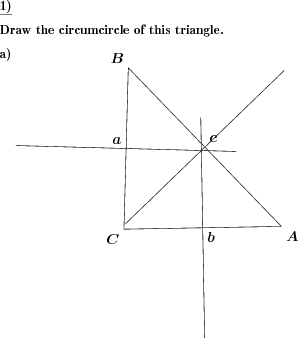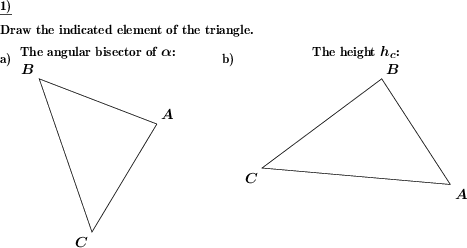Custom math worksheets at your fingertips# Details for problem "Triangle draw incircle"

Quickname: 9755

Junior High School, Middle School, High School.

## Summary

The incircle and angle bisectors have to be drawn for a given triangle.

## Examples## Description

The task is to draw the incircle of a given triangle. To achieve this, the angle bisectors have to be constructed.

The triangle can be presented as a drawing, or it may be necessary to draw it first. In the latter case, some dimensions are given that unambiguously specify the triangle.

The dimensions given can be chosen to be either

• the lengths of the three sides
• the site of two angles and the length of the common side
• the length of two sides and the size of the angle between these sides
• or a random selection of the three possibilities above.

It can be chosen whether the angle bisectors are included in the given triangle, or have to be constructed. In the latter case, there may be a hint in the task description that mentions the necessity of the angle bisectors, or this hint may be omitted.

Download free printable worksheets for this math problem here. The worksheet contains the problems only, the solution sheet includes the answers. Just click on the respective link.

•Worksheet 1Solution sheet with answers
•Worksheet 2Solution sheet with answers
•Worksheet 3Solution sheet with answers

If you can not see the solution sheets for download, they may be filtered out by an ad blocker that you may have installed. If this is the case, please allow ads for this page and reload the page. The solution sheets will then reappear.

• Do these sample worksheets do not really fit?
• Do you need more math worksheets, with a different level of difficulty?
• Would you like to combine different problems on a worksheet and adjust them to your needs?
• As a teacher, you can put together your own worksheets using the automatically generated math problems provided.
With a free initial credit, you can start creating your own math worksheets in a few minutes.

You can try it for free! Register here, to create custom worksheets now!

## Customization options for this problem

Parameter
Possible values
Number of problems
1, 2
Given
sides, angle,side,angle, side,angle,side, sss or asa, sss or sas, asa or sas, sss, sas or asa, triangle w/o a.bisectors, triangle w/ a.bisectors
Mention angle bisectors
Yes, No

## Similar problems

Remark
Description
Angle bisectors in a triangle, no reference to incircle
Draw heights, medians, angle bisectors or perpendicular bisectors for a triangle.

## dw-Math worksheet templates that do contain this problem

Name
Title
Description
Label advanced parts of a triangle
Construct perpendicular bisectors, angle bisectors and medians.

## Other types of problems that appear on worksheets with this problem:

Relevance
Name
Description
Quickname
Example
****
Triangle mark centroid
The medians and centroid have to be drawn for a given triangle.****
Triangle draw circumcircle
The circumcircle and perpendicular bisectors have to be drawn for a given triangle.**
Triangle: Draw heights, medians and bisectors
Draw heights, medians, angle bisectors or perpendicular bisectors for a triangle.**
Angle types
For a given angle, the correct type has to be marked.Deutsche Version dieser Aufgabe
These informational pages with samples describe math problems that can be combined on custom math worksheets with solutions for home and K-12 school use.
Deutsche Seiten
×